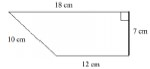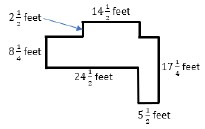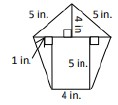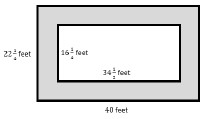# MA.6.GR.2.2Export Print
Solve mathematical and real-world problems involving the area of quadrilaterals and composite figures by decomposing them into triangles or rectangles.

### Clarifications

Clarification 1: Problem types include finding area of composite shapes and determining missing dimensions.

Clarification 2: Within this benchmark, the expectation is to know from memory a formula for the area of a rectangle and triangle.

Clarification 3: Dimensions are limited to positive rational numbers.

General Information
Subject Area: Mathematics (B.E.S.T.)
Strand: Geometric Reasoning
Status: State Board Approved

## Benchmark Instructional Guide

• Algorithm
• Area
• Rectangle
• Triangle

### Vertical Alignment

Previous Benchmarks

Next Benchmarks

### Purpose and Instructional Strategies

Students will use their understanding from grade 5 of finding the perimeter and area of rectangles with fractional or decimal sides to finding the areas of quadrilaterals and composite figures with positive rational number side lengths in grade 6 (MTR.1.1, MTR.2.1). Students will extend this knowledge in grade 7 to decompose composite figures into triangles and quadrilaterals in order to find area.
• Instruction includes finding missing dimensions with quadrilaterals and composite figures (MTR.1.1, MTR.5.1).
• Instruction includes representing measurements for area as square units, units squared or units2.
• When using rational numbers, instruction is restricted to numbers within the same form. Students should not be penalized though if they convert from one form to another when performing operations.
• For example, if students are working with fractions, the side lengths will not include decimals. If students are working with decimals, the side lengths will not include fractions.
• Students should look for opportunities to either decompose or compose shapes to enhance their geometric reasoning.
• For example, in the diagram below, students can solve by decomposing or by composing.Area = (18·7)− $\frac{\text{1}}{\text{2}}$(6·7) = 105 cm2
Area = (12·7)+ $\frac{\text{1}}{\text{2}}$(6·7) = 105 cm2
• Problem types include having students measure lengths using a ruler to determine the area.

### Common Misconceptions or Errors

• Students may invert the perimeter and area formulas.
• Students may incorrectly label all sides of the figure.
• Students may incorrectly identify a side of a triangle as the height.

### Strategies to Support Tiered Instruction

• Teacher reviews the definitions of surface area and volume, and co-creates an anchor chart to display in the room explaining each. Providing flash cards or cue cards with the formulas will help students in place of anchor charts when they are outside the classroom area.
• Teacher models the use of different color markers or pencils to match similar sides when decomposing figures. This will help student accurately label the sides of each shape.
• Use manipulatives that students can measure to better understand there is a difference between a side length and the height in non-right triangles.
• Teacher models the use of manipulatives and geometric software to review the concept of perimeter or area.
• Teacher models the use of manipulatives shapes to reinforce the sides of the pieces that make up a decomposed figure.
• Teacher models the use of manipulatives that students can measure to better understand there is a difference between a side length and the height in non-right triangles.

Instructional Task 1 (MTR.1.1, MTR.2.1, MTR.5.1)
The diagram shows the dimensions, in feet, of the local playground. While playing with friends, Shona lost the key to her diary somewhere in the dirt. By composing or decomposing into rectangles, determine the maximum number of square feet that Shona may need to search to find the missing key? If she only searched one rectangular area, what is the least number of square feet Shona searched?### Instructional Items

Instructional Item 1
A pentagon is shown. What is the area, in square inches, of the pentagon? Image not to scale.Instructional Item 2
Mr. Moretti wants to cover the walkway around his swimming pool with tile. Determine how many square feet of tile he will need to cover the shaded portion of the diagram.*The strategies, tasks and items included in the B1G-M are examples and should not be considered comprehensive.

## Related Courses

This benchmark is part of these courses.
1200400: Foundational Skills in Mathematics 9-12 (Specifically in versions: 2014 - 2015, 2015 - 2022, 2022 and beyond (current))
1205010: M/J Grade 6 Mathematics (Specifically in versions: 2014 - 2015, 2015 - 2022, 2022 and beyond (current))
1205020: M/J Accelerated Mathematics Grade 6 (Specifically in versions: 2014 - 2015, 2015 - 2020, 2020 - 2022, 2022 and beyond (current))
1204000: M/J Foundational Skills in Mathematics 6-8 (Specifically in versions: 2014 - 2015, 2015 - 2022, 2022 and beyond (current))
7812015: Access M/J Grade 6 Mathematics (Specifically in versions: 2014 - 2015, 2015 - 2018, 2018 - 2022, 2022 and beyond (current))

## Related Access Points

Alternate version of this benchmark for students with significant cognitive disabilities.
MA.6.GR.2.AP.2: Decompose quadrilaterals and composite figures into simple shapes (rectangles or triangles) to measure area.

## Related Resources

Vetted resources educators can use to teach the concepts and skills in this benchmark.

## Formative Assessments

Swimming Pool Walkway:

Students are asked to solve a problem involving finding the area of a composite plane figure.

Type: Formative Assessment

Lost Key:

Students are asked to find the area of a composite plane figure.

Type: Formative Assessment

Students are asked to find the area of a trapezoid and a parallelogram by composing or decomposing into triangles and rectangles.

Type: Formative Assessment

Area of Kite:

Students are asked to find the area of a kite by composing it into rectangles or decomposing it into triangles.

Type: Formative Assessment

## Lesson Plans

Using Nets to Find the Surface Area of Pyramids:

In this lesson, students will explore and apply the use of nets to find the surface area of pyramids.

Type: Lesson Plan

Area of a Triangle:

This lesson is primarily formative in nature and is designed to introduce students to the area of a triangle by having them derive the formula themselves using the relationship between rectangles and triangles. During the lesson the teacher will be facilitating their students as they work with their teams and shoulder partners to solve problems.

Type: Lesson Plan

Enrique's Ruined Carpet:

In this activity, students use a house blueprint to find the area of carpeted floor by decomposing composite shapes into rectangles and triangles. As students critique each other's reasoning, they refine their thinking of surface area.

Type: Lesson Plan

Wallpaper Woes Money Math: Lessons for Life:

Students hear a story about a middle-school student who wants to redecorate his bedroom. They measure the classroom wall dimensions, draw a scale model, and incorporate measurements for windows and doors to determine the area that could be covered by wallpaper. Students then hear more about the student's redecorating adventure and learn about expenses, budget constraints, and tradeoffs.

Type: Lesson Plan

## Original Student Tutorial

Area of Triangles: Missing Dimensions:

Follow George as he calculates the missing values for the base and height of triangles in this interactive tutorial.

Type: Original Student Tutorial

## Tutorials

Volume through Decomposition:

This Khan Academy tutorial video illustrates how to find the volume of an irregular solid figure by dividing the figure into two rectangular prisms and finding the volume of each.  Although the tutorial works from a drawing, individual volume cubes are not drawn so students must work from the formula.

Type: Tutorial

Volume: Decomposing a Solid Figure Example:

This Khan Academy tutorial video illustrates finding the volume of an irregular figure made up of unit cubes by separating the figure into two rectangular prisms and finding the volume of each part.

Type: Tutorial

Finding Area by Decomposing a Shape:

This tutorial demonstrates how the area of an irregular geometric shape may be determined by decomposition to smaller familiar shapes.

Type: Tutorial

## MFAS Formative Assessments

Area of Kite:

Students are asked to find the area of a kite by composing it into rectangles or decomposing it into triangles.

Students are asked to find the area of a trapezoid and a parallelogram by composing or decomposing into triangles and rectangles.

Lost Key:

Students are asked to find the area of a composite plane figure.

Swimming Pool Walkway:

Students are asked to solve a problem involving finding the area of a composite plane figure.

## Original Student Tutorials Mathematics - Grades 6-8

Area of Triangles: Missing Dimensions:

Follow George as he calculates the missing values for the base and height of triangles in this interactive tutorial.

## Student Resources

Vetted resources students can use to learn the concepts and skills in this benchmark.

## Original Student Tutorial

Area of Triangles: Missing Dimensions:

Follow George as he calculates the missing values for the base and height of triangles in this interactive tutorial.

Type: Original Student Tutorial

## Tutorials

Volume through Decomposition:

This Khan Academy tutorial video illustrates how to find the volume of an irregular solid figure by dividing the figure into two rectangular prisms and finding the volume of each.  Although the tutorial works from a drawing, individual volume cubes are not drawn so students must work from the formula.

Type: Tutorial

Volume: Decomposing a Solid Figure Example:

This Khan Academy tutorial video illustrates finding the volume of an irregular figure made up of unit cubes by separating the figure into two rectangular prisms and finding the volume of each part.

Type: Tutorial

Finding Area by Decomposing a Shape:

This tutorial demonstrates how the area of an irregular geometric shape may be determined by decomposition to smaller familiar shapes.

Type: Tutorial

## Parent Resources

Vetted resources caregivers can use to help students learn the concepts and skills in this benchmark.